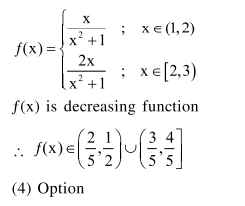# Prove the following

Question:

Let $f:(1,3) \rightarrow \mathrm{R}$ be a function defined by $f(\mathrm{x})=\frac{\mathrm{x}[\mathrm{x}]}{1+\mathrm{x}^{2}}$, where $[\mathrm{x}]$ denotes the greatest integer $\leq \mathrm{x}$. Then the range of $f$ is

1. $\left(\frac{3}{5}, \frac{4}{5}\right)$

2. $\left(\frac{2}{5}, \frac{3}{5}\right] \cup\left(\frac{3}{4}, \frac{4}{5}\right)$

3. $\left(\frac{2}{5}, \frac{4}{5}\right]$

4. $\left(\frac{2}{5}, \frac{1}{2}\right) \cup\left(\frac{3}{5}, \frac{4}{5}\right]$

Correct Option: , 4

Solution: